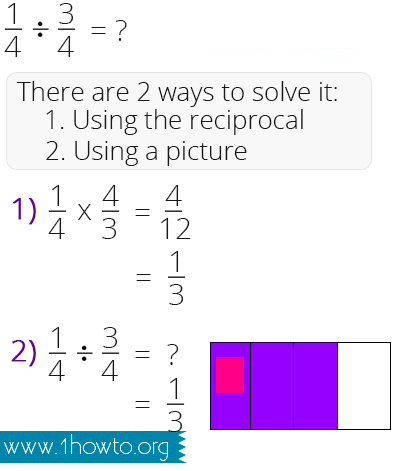﻿ How to Divide Fractions
www.1howmany.com
Education & Reference / How to Divide Fractions

# How to Divide Fractions

Dividing a whole number by a whole number is usually not a very difficult task, and almost everybody knows how to do it. But when it is necessary to divide fractions1, it can be a thorny problem for many people. For instance, do you know how to solve something like this: 3/5 ÷ 4/9? Do not feel ashamed, if you cannot do it. Keep reading this article and we will teach you how to divide fractions.

In fact, there are several ways of dividing fractions and we will explain you the two easiest ones.

Inverting and Multiplying

In order to divide fractions, using this method, do the following:

• First of all, you have to invert the fraction (just flip it over) of the divisor. You will get what is called "the reciprocal"2. E.g. 2/5 becomes 5/2.
• Multiply the fractions. We hope you know how to multiply fractions. All you have to do is to multiply the denominators3 and numerators4.
• If possible, convert them to a mixed number. E.g. 13/8 will be 1 5/8.

For example: 2/3÷2/5 = 2/3*5/2 = 10/6 = 1 4/6 = 1 2/3Common Denominator

Another way of dividing fractions is using a common denominator. This method is lengthier but it can be more useful in everyday life. It includes the following steps:

• You should find a common denominator. Then you need to convert the fractions to the equivalent value.
• Now then the denominators are equal it is possible to divide the numerators.

E.g.: 4/5÷2/3 = 12/15÷10/15 = 12/10 = 1 1/5

Now you know two ways of dividing fractions. If you are going to teach your child how to divide a fraction by a fraction, you should better explain him the first method, because it is really much easier.
You may be interested in: